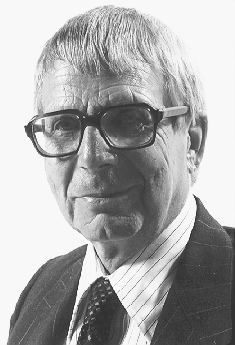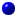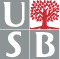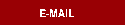GeneralSpeakersScheduleProceedingsRegistrationAccommodationsTransportationRelated EventsAhlfors-Bers Colloquium
Stony Brook 1998SPEAKERSFRANCIS BONAHON - University of Southern California
Bending Measured Laminations of Hyperbolic 3-Manifolds

Abstract:   (joint work with Jean-Pierre Otal)
Let M be a compact 3-manifold with non-empty boundary. If the topological necessary conditions are satisfied, the hyperbolization theorem of Thurston and the Ahlfors-Bers double uniformization theorem provide many geometrically finite hyperbolic structures on the interior of M. An invariant of such a geometrically finite hyperbolic structure is the bending measured lamination of its convex core, which is a measured geodesic lamination on the boundary of M. We will consider the inverse problem of characterizing which measured geodesic laminations on the boundary of M can be realized as bending measured laminations of geometrically finite hyperbolic structures on the interior of M. The problem is completely solved when M has incompressible boundary. The problem is much more difficult when the boundary of M is compressible, and we will discuss some additional necessary (conjecturally sufficient?) conditions in this case.LENNART CARLESON - Mittag-Leffler Institute
The Hexagonal Lattice in Complex AnalysisDARYL COOPER - U. C. Santa Barbara
The Orbifold Theorem

Abstract: The proof of Thurston's Orbifold theorem requires a Margulis type theorem describing the thin part of a hyperbolic 3-manifold with cone-type singularities. This requires the classification of non-compact Euclidean cone-manifolds. We will describe a strong version of the Soul Theorem for these objects.HERSHEL FARKAS - Hebrew University of Jerusalem
Riemann Surfaces and Theta FunctionsBRIAN GREENE - Columbia University
Exotic Properties of Spacetime in String TheoryPHILLIP A. GRIFFITHS - Institute for Advanced Study
K2(C) and Complex Function Theory

Abstract:     The study of meromorphic functions on a compact Riemann surface leads to the Jacobian variety, which is the fundamental invariant of the Riemann surface. For a triangle viewed as a degenerate Riemann surface of genus one, the Jacobian variety becomes C*, which to algebraists is known as the group K1(C). For a complex algebraic structure the analogue of the Jacobian variety is not understood and presents one of the most fundamental challenges to Hodge theory. However, for the tetrahedron viewed as a degenerate quartic surface -the analogue for surfaces of genus one curves- the Jacobian can be constructed and it turns out to be the group K2(C).EMILY HAMILTON - Emory University
Geometrical Finiteness for Hyperbolic OrbifoldsDENNIS HEJHAL - University of Minnesota and Uppsala
Kernel Functions, Poincaré Series, and LVANIKOLA LAKIC - Cornell University
Asymptotic Teichmüller SpaceFENG LUO - Rutgers University
SL(2,C) Representations of the Surface Groups

Abstract:     Each hyperbolic metric on a surface can be constructed as follows. Assign to each subsurface homeomorphic to a 1-holed torus or a 4-holed sphere a hyperbolic metric so that when two such subsurfaces overlap in an annulus or a 3-holed sphere, they overlap geometrically. The hyperbolic metric on the surface is constructed as the result of gluing of all hyperbolic metrics on the subsurfaces. This principle of reconstruction is extended to SL(2,C) linear representations (and their characters) of the fundamental groups of the surfaces.YAIR MINSKY - S.U.N.Y. at Stony Brook
Kleinian Groups and the Complex of CurvesSCOTT A. WOLPERT - University of Maryland
Ramanujan, Coefficient Sums and Automorphic Quantum ErgodicityTentative list, subject to change. © 1998. Department of Mathematics, S.U.N.Y., Stony Brook.